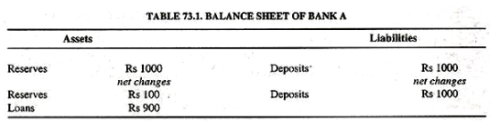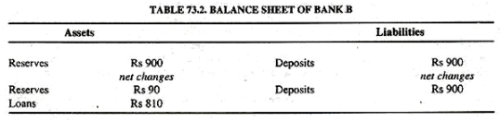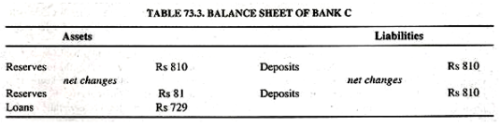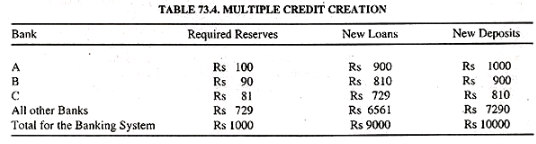Courses

# Process of Credit Creation, Indian Financial System B Com Notes | EduRev

## B Com : Process of Credit Creation, Indian Financial System B Com Notes | EduRev

The document Process of Credit Creation, Indian Financial System B Com Notes | EduRev is a part of the B Com Course Indian Financial System.
All you need of B Com at this link: B Com

Credit Creation : The Process of Credit Creation in Commercial Banks

Let us explain the actual process of credit creation. The ability of banks to create credit depends on the fact that banks need only a small percentage of cash to deposits. If banks kept 100 per cent cash against deposits, there would be no credit creation. Modern banks do not keep 100 percent cash reserves.

They are legally required to keep a fixed percentage of their deposits in cash, say 10, 15 or 20 per cent. They lend and/or invest the remaining amount which is called excess reserves. A bank can lend equal to its excess reserves. But the entire banking system can lend and create credit (or deposits) upto a multiple of its original excess reserves. The deposit multiplier depends upon the required reserve which is the basis of credit creation. Symbolically, the required reserve ratio:

RRr = RR/D

or RR = RRr x D

Where RR are the required cash reserves with banks, RRr is the required reserve ratio and D is the demand deposits of banks. To show that D depends on RR and RRr, divide both sides of the above equation by RRr:

RR/RRr = RRr x D/RRe

Or RR/RRr = D

Or 1/RRr = D/RR

Or D = 1/RRr = x RR

Where 1/RRr, the reciprocal of the percentage reserve ratio, is called the deposit (or credit) expansion; the limits of the deposit expansion of a bank. The maximum amount of demand deposits which the banking system can support with any given amount of RR is by applying the multiplier to RR. Taking the initial change in the volume of deposits (DD) and in cash reserves (DRR), it follows from any given percentage of RRr that

∆D = RR x 1/RRr

To understand it, suppose the RRr for the banks is fixed at 10 per cent and the initial change in cash reserves is Rs 1000. By applying the above formula, the maximum increase in demand deposits will be

∆D = 1000 x 1/0.10 = Rs. 10000.

This is the extent to which the banking system can create credit. The above equation can also be expressed as follows:

DD = RR [1+(1-RRr) + (Y-RRr)2+…+(1-RRr)n]

The sum of the geometric progression within brackets gives:

1/1-(1 – RRr) = 1/RRr

∆D = ∆RR x 1/RRr

The deposit expansion multiplier rests on the assumptions that banks lend out all their excess reserves and RRr remains constant.

To explain the process of credit creation, we make the following assumptions:

1. There are many banks, say А, В, C, etc., in the banking system.

2. Each bank has to keep 10 percent of its deposits in reserves. In other word 10 per cent is the required ratio fixed by law.

3. The first bank has Rs. 1000 as deposits.

4. The loan amount drawn by the customer of one bank is deposited in full in the second bank, and that of the second bank into the third bank, and so on.

5. Each bank starts with the initial deposit which is deposited by the debtor of the other bank.

Given these assumptions suppose that Bank A receives cash deposits of Rs. 1000 to begin with. This is the cash in hand with the bank which is its asset and this amount is also the liability of the bank by way of deposits it holds. Given the reserve ratio of 10 per cent, the bank keeps Rs. 100 in reserves and lends Rs 900 to one of its customers who, in turn, give a cheque to some person from whom he borrows or buys something. The net changes in Bank A’ is balance sheet are +Rs 100 in reserves and +Rs 900 in loans on the assets side and Rs 1000 in demand deposits on the liabilities side as shown in Table 73.1. Before these changes Bank A had zero excess reserves.This loan of Rs. 900 is deposited by the customer in Bank B whose balance sheet is shown in Table 73.2. Bank B starts with a deposit of Rs. 900, Keeps 10 per cent of it or Rs. 90 as cash in reserve. Bank B has Rs 810 as excess reserves which it lends thereby creating new deposits.This loan of Rs. 810 is deposited by the customer of Bank B into Bank C. The balance sheet of Bank C is shown in Table 73.3. Bank C keeps Rs 81 or 10 per cent of Rs 810 in cash reserves and lends Rs. 729.This Process goes on to other banks. Each bank in the sequence gets excess reserves, lends and creates new demand deposits equal to 90% of the preceding bank’s. In this way, new deposits are created to the tune of Rs. 10000 in the banking system, as shown in Table 73.4.The multiple credit creation shown in the last column of the above Table can also be worked out algebraically as:

Rs 1000[1+(9/10)+(9/10)2+(9/10)3+…+(9/10)”]

=Rs 1000(1/1-9/10)= Rs 1000(1/1/10)= Rs 1000×10 = Rs 10000.

Offer running on EduRev: Apply code STAYHOME200 to get INR 200 off on our premium plan EduRev Infinity!

## Indian Financial System

38 videos|39 docs

,

,

,

,

,

,

,

,

,

,

,

,

,

,

,

,

,

,

,

,

,

,

,

,

;